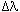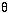We are here with you hands in hands to facilitate your learning & don't appreciate the idea of copying or replicating solutions. Read More>>

#www.vustudents.ning.com

 www.bit.ly/vucodes + Link For Assignments, GDBs & Online Quizzes Solution www.bit.ly/papersvu + Link For Past Papers, Solved MCQs, Short Notes & More

Dear Students! Share your Assignments / GDBs / Quizzes files as you receive in your LMS, So it can be discussed/solved timely. Add Discussion

Graded Discussion Board (GDB) for the course PHY101 (PHYSICS) will start on Thursday 04th August, 2016.

You should remember that you have to post your comments about the discussion title within the time.

This discussion board will be closed on Friday 05th August, 2016.

No comment about the Discussion topic should be posted on the regular Moderated Discussion Board which is created for each lecture.

The topic for the discussion is:

“Justify the following statement
If you calculate the speed of light from a star that is moving toward you, you will get a higher value than if you calculate the speed of light from a star that is moving away from you?
Either yes or no, give a solid reason for its justification”
Elaborate your answer by giving a solid reason, give mathematical calculation (proof) where necessary.

Also you should remember that this Discussion is graded and maximum marks for this Graded Discussion Board 5% and it will count in your final Grade. So these marks may be helpful for you to get A grade from B and B grade from C and so on.

In the end you should also know that we will not extend the date of discussion in any case.

Plagiarism is prohibited that is why you are advised to avoid copy/paste from internet etc, if found at any stage will be graded as zero marks.
· All students are directed to use the font face Time new Roman and Font size 12.

Don’t use colorful back grounds in your solution.
Use Math Type or Equation Editor etc for mathematical symbols.
It also suggests you to keep your GDB solution safe from others. No excuse will be accepted by anyone if found to be copying or letting others copy.

+ How to Join Subject Study Groups & Get Helping Material?

+ How to become Top Reputation, Angels, Intellectual, Featured Members & Moderators?

+ VU Students Reserves The Right to Delete Your Profile, If?

Views: 1781

.

+ http://bit.ly/vucodes (Link for Assignments, GDBs & Online Quizzes Solution)

+ http://bit.ly/papersvu (Link for Past Papers, Solved MCQs, Short Notes & More)

### Replies to This Discussion

kesi ko nahi ata kiya

'IDEA.

Attachments:plzz explain it

kya is me doppler effect istamal hota he.........................?

Justify the following statement

If you calculate the speed of light from a star that is moving toward you, you will get a higher value than if you calculate the speed of light from a star that is moving away from you?

Either yes or no, give a solid reason for its justification

It has nothing to do with math, but you couldn't measure the speed of a star travelling away from you. A lot of what we see in the night sky is actually entire galaxies containing billions of individual stars, concentrated into individual points of light due to distance. The star Cassiopeia is 4,000 light years away from earth and is the furthest star (and it is still in our galaxy) visible with the naked eye. Therefore, no stars that are visible to us travel away from us at the speed of light

Light always travels at the speed of light within a reference frame. This is one of the tenets of relativity theory, and has been justified by measurement.

What happens is that if the source is moving away, it's frequency will be lower than emitted by the source (redshift), or if it is moving toward, the frequency will be higher (blueshift). The speed of the light does not change

The velocity of stars are determined by using the doppler effect (see the doppler effect section). The motion of a star causes a shift in the wavelengths received. The star emits the same spectrum it would emit if it was at rest. The pattern of lines depends on the internal physics of the star itself. The doppler effect depends on the external property of the star's motion through space relative to us. The wavelength shift=new -rest, wherenew is the observed wavelength andrest is the wavelength you would observe if the star was not moving relative to us. The amount of the shift depends on the velocity of the star in this way:rest = (radial velocity) / c, where c is the speed of light and the radial velocity is the component of the star's motion that is along the line of sight. Rearranging the terms you find the radial velocity = (rest) × c.

If the star is moving away from you, then you see the wavelengths stretched out andis a positive number. The spectral lines appear shifted to the red end of the spectrum, so the shift is called a redshift. If the star is moving toward you, then the wavelengths appear to the compressed andis a negative number. The spectral lines are shifted to the blue end of the spectrum, so the shift is called a blueshift. The doppler effect tells you only about the motion relative to you. If you move toward the star or it moves toward you, the doppler effect will be the same. The Earth is in motion relative to the star because it are orbiting the Sun, so you have to take the Earth's orbital motion into account when figuring the star's velocity from the doppler effect.

Also, the doppler effect gives you only the speed along the line of sight. Most stars move at an angle to our line of sight. The part of a star's total velocity that is perpendicular to the line of sight is called the tangential velocity.

To get the tangential velocity, you need to first measure the angular velocity of the star across the sky (d/dt). This is how many degrees on the sky the star moves in a given amount of time and is called the proper motion by astronomers. If you determine the star's distance from its trigonometric parallax or the inverse square law method, you can convert the angular velocity (proper motion) to tangential velocity in physical units such as kilometers/second. The tangential velocity = k × the star's distance × the proper motion, where k is a conversion factor that will take care of the conversion from arc seconds and parsecs and years to kilometers/second. Using the Pythagorean theorem for right triangles, you find that the star's total velocity = Sqrt[(radial velocity)2 + (tangential velocity)2].

.